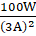Solve the example. Heat energy is being produced in a resistance in a circuit at the rate of 100 W. The current of 3 A is flowing in the circuit. What must be the value of the resistance?

Question 16.

Solve the example.

Heat energy is being produced in a resistance in a circuit at the rate of 100 W. The current of 3 A is flowing in the circuit. What must be the value of the resistance?

Answer: Given Power P = 100 W

Current I = 3 A

R =?

We know P = I2R;

where R is resistance

So R = P/ I2

⇒, R == 11.11 ohm